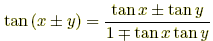distributing rules

Distributing Rules

Algebra rules for distributingexpressions. See factoring rules as well.

 A. Multiplication1. addition:  a(b + c) = ab + ac  and  (b + c)a = ba + ca2. subtraction:  a(b – c) = ab – ac  and  (b – c)a = ba – ca3. FOIL:  (a + b)(c + d) = ac + ad + bc + bdCareful!!a(bc) ≠ ab·ac B. Division1. addition: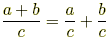2. subtraction: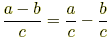Careful!!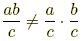C. Exponents   (a ≥ 0, b ≥ 0)1. multiplication:  (ab)x = axbx2. division: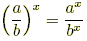(b ≠ 0)Careful!!(a + b)x ≠ ax + bx(a – b)x ≠ ax– bx D. Roots   (x ≥ 0, y ≥ 0)1. multiplication: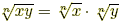2. division: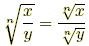( y ≠ 0)Careful!!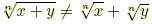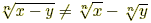E. Logarithms   (x > 0, y > 0, a > 0, a ≠ 1)1. multiplication:  loga (xy) = logax + logay2. division: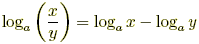3. powers:  loga (xp) = p logaxCareful!!loga (x + y) ≠ logax + logayloga (x – y) ≠ logax– logay F. Trig1.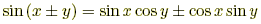2.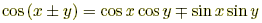3.